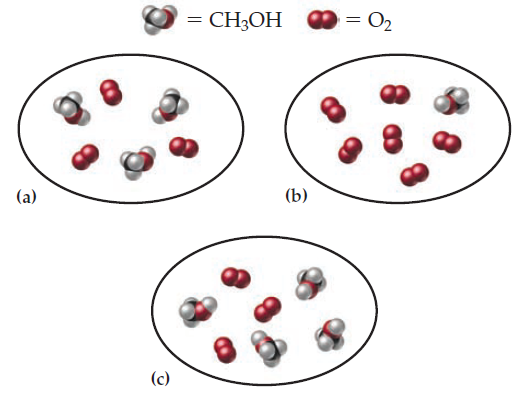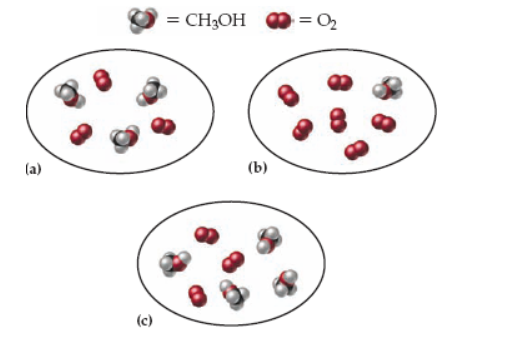×
Get Full Access to Introductory Chemistry - 5 Edition - Chapter 8 - Problem 54p
Get Full Access to Introductory Chemistry - 5 Edition - Chapter 8 - Problem 54p

×

# Consider the reaction:2 CH3OH(g) + 3 O2(g) ? 2 CO2(g) + 4 H2O(g)Each molecular diagramISBN: 9780321910295 34

## Solution for problem 54P Chapter 8

Introductory Chemistry | 5th Edition

• Textbook Solutions
• 2901 Step-by-step solutions solved by professors and subject experts
• Get 24/7 help from StudySoup virtual teaching assistantsIntroductory Chemistry | 5th Edition

4 5 1 264 Reviews
13
5
Problem 54P

Consider the reaction:

2 CH3OH(𝘨) + 3 O2(𝘨)¡2 CO2(𝘨) + 4 H2O(𝘨)

Each molecular diagram represents an initial mixture of the reactants. How many CO2 molecules are formed by complete reaction in each case? (Assume 100% actual yield.)Step-by-Step Solution:

PROBLEM 54P

Consider the reaction:

2 CH3OH(g) + 3 O2(g) ? 2 CO2(g) + 4 H2O(g)

Each molecular diagram represents an initial mixture of the reactants. How many CO2 molecules are formed by complete reaction in each case? (Assume 100% actual yield.)Step by step solution

Step 1 of 3

a)

There are three methanol molecules and 3 oxygen molecules, as we can see.

Oxygen is limiting here and will fully react to form two carbon dioxide molecules.

___________________________________________________________________________

Step 2 of 3

Step 3 of 3

##### ISBN: 9780321910295

Unlock Textbook Solution